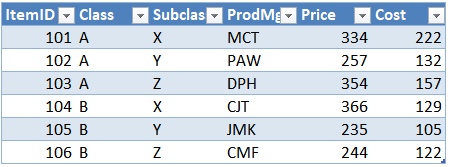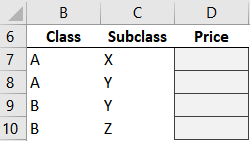## Battle of Heavyweights: Final Analysis

In this, our final analysis, we will briefly recap each of the 8 rounds and confirm we understand the key differences between these two incredible functions. Then, we’ll confirm we know when and how to apply each in practice. Let’s get to it.## Winner?

So, which function is better? Which one should we always use? Who is the winner? The goal of this series was to explore the differences between these two incredible functions so that you are comfortable knowing when to use each. The boxing metaphor was used as a structure to organize the presentation, and I hope it was a fun way to accomplish the goal 🙂

These functions are designed to do different things. But, since there is overlap between their capabilities, I often hear students ask “Since they can both do similar things, which one should I use?” And this question was the motivation for the series.

I didn’t write this series to declare a winner! I wrote it to provide a fun way to present and explore the details of these two champions. They are both mission-critical Excel skills and you’ll use them both in practice. I hope that this series has helped you understand their differences, capabilities, details and feel like you know when to use each one.

So, we’ll recap the differences and then comes the moment of truth. We’ll see if you are comfortable determining which to use in three scenarios.

## Recap

Here is a brief recap of each of the rounds:

1. When there are multiple matching rows:
• VLOOKUP stops at the first match
• SUMIFS returns the sum of all matching rows
2. When equivalent values are stored as different data types:
• VLOOKUP does not match them
• SUMIFS does match them
3. Is the column order important?
• VLOOKUP assumes the lookup column is the first column in the lookup range
• SUMIFS doesn’t care about column order
4. Return text values?
• VLOOKUP can return text or numbers
• SUMIFS is designed to return a sum, a number (not text values)
5. What if there are no matching rows?
• VLOOKUP TRUE uses approximate match
• VLOOKUP FALSE returns an error
• SUMIFS returns 0
6. When we insert a new worksheet column between the lookup and return columns:
• VLOOKUP will break when the 3rd argument is expressed as a whole number
• SUMIFS doesn’t break
7. Multiple lookup columns?
• VLOOKUP supports a single lookup column
• SUMIFS handles multiple lookup columns
8. Can we do a range lookup?
• VLOOKUP TRUE is designed to perform a range lookup, and operates with a single lookup column
• SUMIFS can perform a range lookup using concatenation when both sides of each range are provided

So, Jeff … just give me a summary statement! I need a simple way to remember when to use each. No problem. In general, here is how I think about it:

If the value I am trying to return is a number, I prefer SUMIFS; otherwise, I use VLOOKUP.

So, you think you got it? Let’s find out. Here are three applications to confirm we are good.

## Applications

We will use the same data table for each of our three applications. I will then provide a screenshot of our little report and identify which value we are trying to retrieve. We will then need to determine which of our two competitors we would use.

First, our data table (Table1):A few things to note. Each item has a unique numeric ItemID (101, 102, …). Each item has Class and Subclass values. When Class and Subclass values are combined, they are unique. That is, no two product share the same class/subclass combination. Each product has a Product Manager (ProdMgr). And each item has a Price that we charge customers, and a Cost we pay suppliers.

So, let’s do our three applications.

### Application 1

Here is our little report:Now, it is our job to reason through this to figure out which of our two competitors to use. Let’s start by looking at the return value, ProdMgr. ProdMgr is a text string. So, which can we use? Well, VLOOKUP can return text strings, so we could use VLOOKUP. What about SUMIFS? SUMIFS returns the sum of all matching rows, it returns a number. Since we are trying to retrieve a text string we’ll go with VLOOKUP.

We write the following formula in C7:

`=VLOOKUP(B7, Table1, 4, 0)`

And fill it down:We look good.

### Application 2

Now, let’s say that this is the report:Which function should we use? Let’s think about it. We want to retrieve Price. As we can see by examining the data table, Price is a number. So, at this point, we could use either VLOOKUP or SUMIFS because both can return a number. So far so good.

But, this time, we don’t have the unique ItemID. All we have are the Class and Subclass values. So, hmmm … there are multiple lookup columns, not a single lookup column. VLOOKUP is designed to operate on a single lookup column, whereas SUMIFS is designed to support multiple lookup columns (multiple conditions). So, in this case, let’s use SUMIFS.

We write the following formula into D7:

`=SUMIFS(Table1[Price], Table1[Class], B7, Table1[Subclass], C7)`

We fill the formula down and:We got it!

### Application 3

This time, we are trying to build this report:So, which function do we use? Let’s figure it out. We are trying to return ProdMgr, which is a text string. So, clearly we can’t use SUMIFS. So, we decide to use VLOOKUP. But then, we notice that we have multiple lookup columns (Class and Subclass). So, clearly, we can’t use VLOOKUP because it supports a single lookup column. But, we remember that SUMIFS supports multiple lookup columns, so we decide to use SUMIFS. But then we remember that the value we are trying to return is a text string. So we clearly can’t use SUMIFS. And we are in this circular loop, where it seems we clearly can’t use either function.

Argh!

If we clearly can’t use SUMIFS and we clearly can’t use VLOOKUP … what are we supposed to do?

Here’s the thing:

These functions aren’t competitors at all! They are allies that can work together 🙂

Wait, what?! Yes … we can use them together, in the same formula so that each can do its thing. They can help each other out.

That is … we can use VLOOKUP to return the ProdMgr text string. And we can use SUMIFS to tell VLOOKUP the ItemID by matching the multiple lookup values. In other words, SUMIFS will be the first argument for VLOOKUP and provide the ItemID.

We write the following formula into D7:

`=VLOOKUP(SUMIFS(Table1[ItemID],Table1[Class],B7,Table1[Subclass],C7), Table1, 4, 0)`

We fill it down and:Yes … it works!

If you’d like more detail about how to nest these functions together, check out this post which provides detailed step-by-step instructions.

And, we could also tackle this with Power Query. If you’d like more info on that, check out this post which provides the details.

Note: we could also create a single lookup column with concatenation and use VLOOKUP alone.

If you enjoyed this blog series, you may also enjoy digging deeper with my online courses. This  series is based on a lesson from one of my online courses (Undergrad V2).

All of my courses are taught with on-demand lecture videos (captioned), and provide downloadable exercise workbooks so you can practice. After completing the homework, you take a quiz and move to the next lesson. These courses also qualify for CPE credit if you need continuing professional education hours.

I offer two main tracks: Undergraduate and Masters. The Undergraduate curriculum teaches classic Excel topics such as VLOOKUP, SUMIFS, PivotTables, and so on … 80+ topics. The Masters courses teach Power Query, Power Pivot, and VBA Macros. More info here:

Whether or not you decide to enroll into a course, I hope that this blog series helped shed some light on the key differences between VLOOKUP and SUMIFS, and helps you feel more comfortable knowing which function to use in your workbooks!

Sample file: FinalAnalysis.xlsxPosted in ,### Jeff Lenning

I love sharing the things I've learned about Excel, and I built Excel University to help me do that. My motto is: Learn Excel. Work Faster.

### Excel is not what it used to be.

You need the Excel Proficiency Roadmap now. Includes 6 steps for a successful journey, 3 things to avoid, and weekly Excel tips.

### Want to learn Excel?

Access all Undergrad and Masters lessons with a Campus Pass or CPE Pass. Includes on-demand training plus live office hours.

!
!